# Algebraic Fractions Worksheet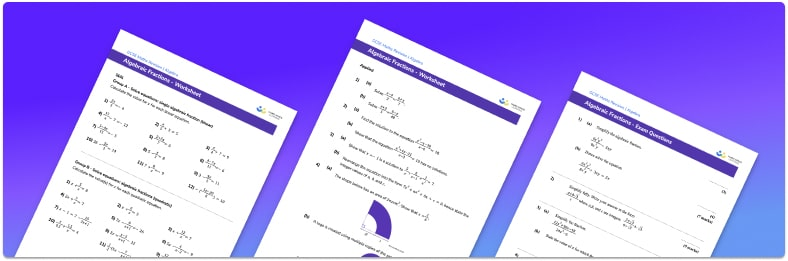• This field is for validation purposes and should be left unchanged.

You can unsubscribe at any time (each email we send will contain an easy way to unsubscribe). To find out more about how we use your data, see our privacy policy.

• Section 1 of the algebraic fractions worksheet contains 36 skills-based factorising questions, in 3 groups to support differentiation
• Section 2 contains 4 applied algebraic fractions questions with a mix of worded problems and deeper problem solving questions
• Section 3 contains 4 foundation and higher level GCSE exam style algebraic fractions questions
• Answers and a mark scheme for all practice questions are provided
• Questions follow variation theory with plenty of opportunities for students to work independently at their own level
• All questions created by fully qualified expert secondary maths teachers
• Suitable for GCSE maths revision for AQA, OCR and Edexcel exam boards

### Algebraic fractions at a glance

Algebraic fractions are fractions which include an algebraic term. The algebraic term may be in its numerator, or denominator or both.

Working with algebraic fractions is a progression from numerical fractions and will help to deepen your understanding of fractions.

For example, equivalent fractions are used to write algebraic fractions in their simplest form by cancelling common factors in the numerator and denominator. Common denominators are also used for adding or subtracting algebraic fractions so that they can then be written as a single algebraic fraction.

Multiplying and dividing are also included as part of algebraic fractions.

When working with algebraic fractions many other algebraic skills are used, such as solving equations, starting with linear equations and moving onto quadratic equations.Looking forward, students can then progress to additional algebra worksheets, for example a straight line graphs worksheet or simultaneous equations worksheet.

For more teaching and learning support on Algebra our GCSE maths lessons provide step by step support and lesson plans for all GCSE maths concepts.

Our maths interventions are currently only available for GCSE students, and are not suitable for A level students.

## Related worksheets

Collecting Like Terms Worksheet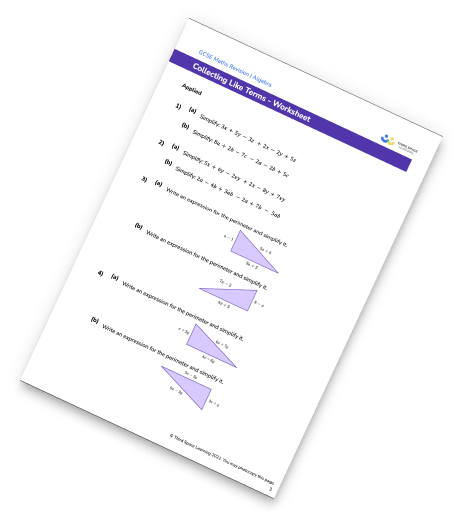Expand and Simplify Worksheet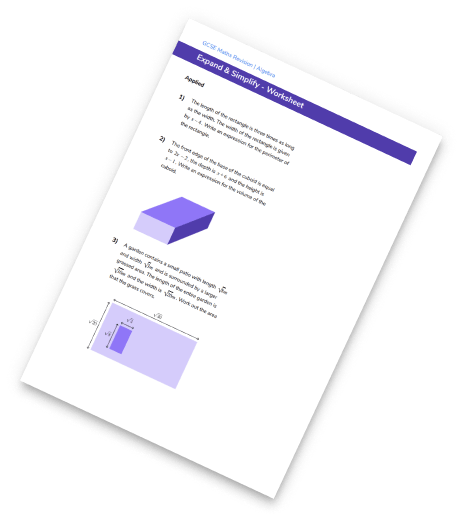Algebraic Expressions Worksheet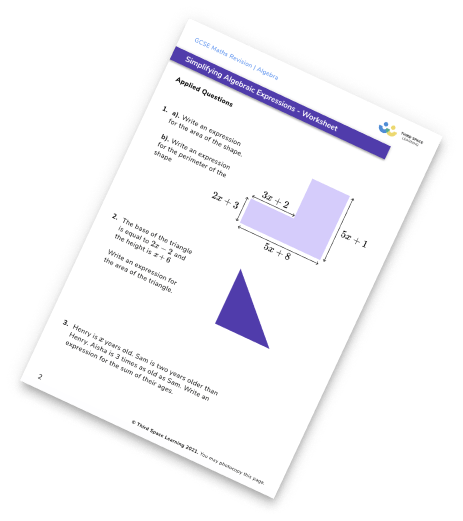Simplifying Expressions Worksheet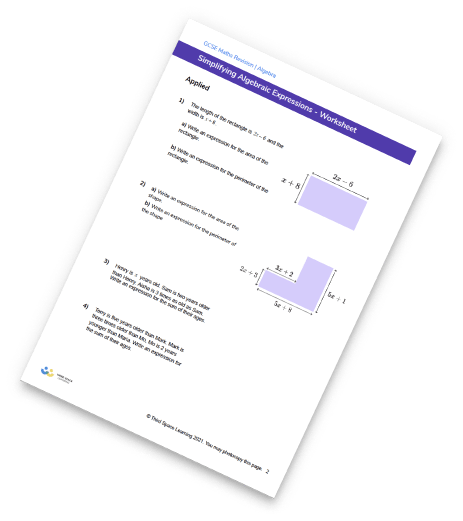## Do you have KS4 students who need more focused attention to succeed at GCSE?There will be students in your class who require individual attention to help them succeed in their maths GCSEs. In a class of 30, it’s not always easy to provide.

Help your students feel confident with exam-style questions and the strategies they’ll need to answer them correctly with our dedicated GCSE maths revision programme.

Lessons are selected to provide support where each student needs it most, and specially-trained GCSE maths tutors adapt the pitch and pace of each lesson. This ensures a personalised revision programme that raises grades and boosts confidence.

Find out more